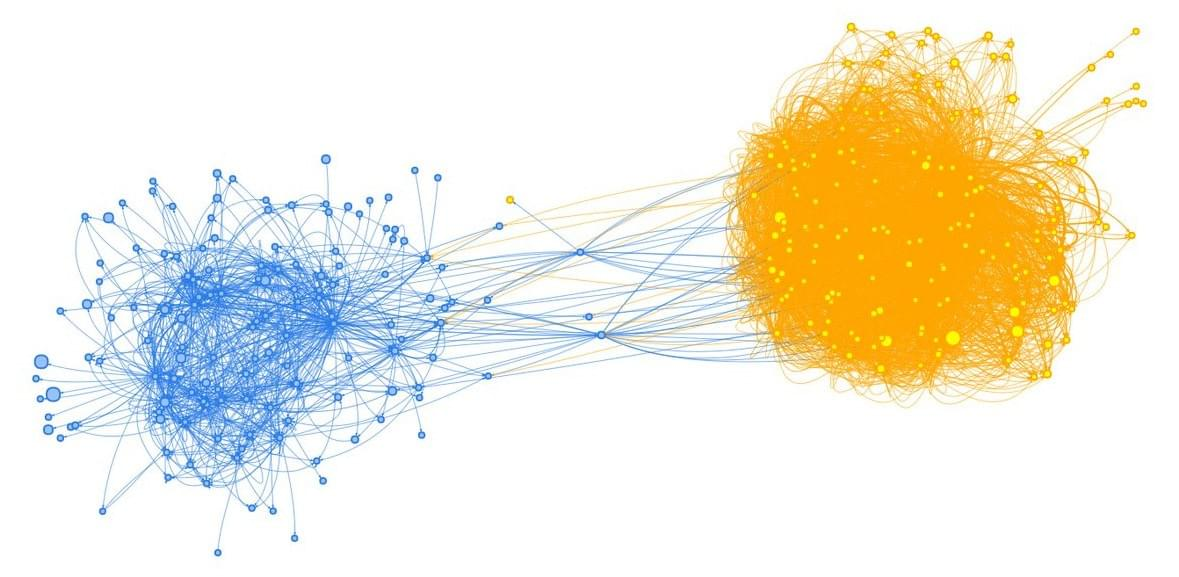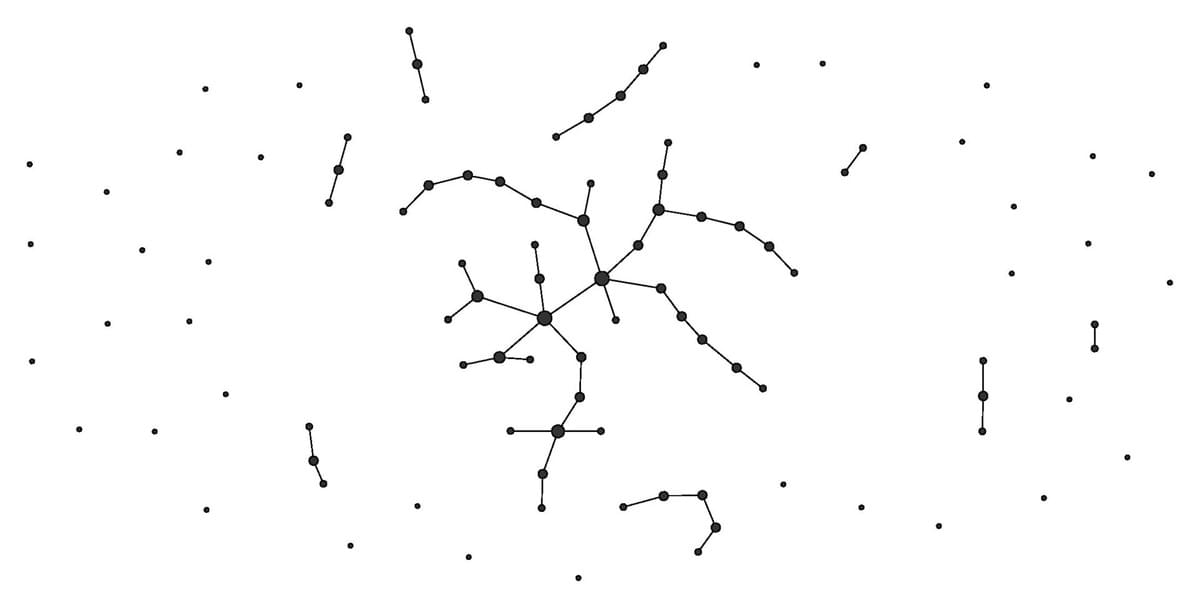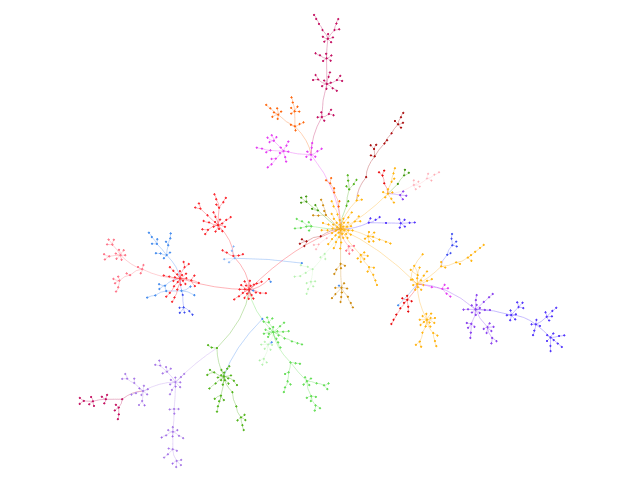# Quick review of graph mining with R

## by Sébastien Loustau

· ToolsAndTech« A graph is a set of objects called vertices, or nodes in which some pairs are in some sense "related" with edges, or lines.» This abstract definition based on Wikipedia shows very well the genericity of a graph and the possible amount of applications. We love graphs because it could be met in many areas. However, the analysis of large networks, ie billions of edges, needs recent technologies and big theory too.

In this article, we propose to overview fundamental models and algorithms to analyze graphs, and focus on the problem of detecting communities in possible large networks. This problem has a wide range of applications and very challenging locks, due to the size of networks observed in practice, like Facebook, LinkedIn, Instagram or Twitter.

Graph models : an overview

Erdõs Rényi random graphs are the first models introduced in the sixties by Paul Erdõs and Alfred Rényi. There are based on the "equally likely" paradigm : in this model, each edge has a fixed probability of being present or absent, independently of the other edges. For instance, any Erdõs Rényi random graph with N vertices and M edges has a same probability to be observed. Since no particular structure is promoted, this model sounds dramatically unrealistic :More recently, the Preferential Attachment Model, or Barabási-Albert model, has been introduced. The model with NN nodes is denoted as PA(N) and is defined recursively as follows : PA(2) is the unique tree with two nodes and PA(N) is given from PA(N−1) by adding a new node j and an edge (j,u) with probability p(u) proportional to the degree of u. Then, a new node has high probability to connect to a node with high degree. This model generates random graphs with properties that have been observed in real-life applications. For instance these models produce scale-free networks: the fraction p(k) of nodes with kkconnexions with other nodes decreases polynomially with k, that is p(k)∼k−γ, where γ>0. In other words, the degree's distribution follows a power law, which means that the fraction of nodes with huge number of edges is not neglictable. These nodes are called "influencer".A Preferential Attachment Model with n~1000

Finally, another more agnostic way to define graphs is to construct similarity-based matrices based on correlations or kernels defined on a classical Euclidean space. In this case, any dataset could be seen as a graph, where nodes are observations and edges are constructed as a function of the similarity (or kernel) matrix K(Xi,Xj).

Graph calculus and storage

A graph database directly uses the graph structure (ie the edges) to relate data items in the store. This contrasts with classical relational databases, such as SQL database where links are stored in the data. By definition, a graph database allows faster queries. However, it depends on the difficulty of the querie. Many different queries can be drawn, such as "Is j connected to i ?", "What is the degree of node k ?", or "What is the shortest path between two nodes ?".
The storage of the graph structure (ie nodes and edges) could be challenging (potentially O(N2)). However in many applications, we deal with sparse graph, where the set of edges evolves linearly with the number of nodes. For this reason, it is more likely to store the edge list instead of the adjacency matrix. Neo4j is the most popular graph database with a large community. It is available under a dual-license arrangement that is business friendly and open-source transparent: the Neo Technology Commercial License (NTCL), or the (A)GPL. Titan is another more recent open source product (Apache 2 license) developed by Aurelius and acquired by Datastax in 2015. It is a scalable and distributed graph database optimized to store large graphs (billions of edges) over several clusters.

Community Detection : code example with R

Graph clustering, or community detection, is usefull for enhanced data exploration and immediate visual insights of large networks. The principle is to detect clusters, with many edges joining vertices of the same cluster and comparatively few edges joining vertices of different clusters. This problem is known to be very hard and has been tackle in many applications, such as biology, social sciences, computer science or more recently social networks. To tackle this problem, spectral approaches have been introduced, inspired from the so-called spectral clustering problem. The principle is to compute the eigen-decomposition of the matrix representation of the graph, the graph Laplacian. However, this approach is computationaly intractable for large networks. The treatment of larger and larger graphs has been investigated and modularity-based algorithms has been proposed. This class of algorithms maximize a quality index called modularity. Unfortunately, exact modularity optimization is NP-hard and becomes also problematic for large networks. With this in mind, approximated solutions based on greedy search has been introduced, such as for instance Louvain algorithm.

Before to give some code to perform both spectral and modularity-based approaches, let us introduce a basic tool for network analysis : Igraph. Igraph is an open-source project programmed in R, python and C/C++. It allows to load, plot and analyze networks easily. In the rest of this post, we will use igraph R package. Here is a simple R script to construct an igraph object and plot a small network with R :

Spectral Clustering with R

Spectral clustering is based on the eigen-decomposition of the Laplacian matrix. Its motivation comes from linear algebra and spectral graph theory. The following R script computes the graph Laplacian of an undirected and weighted graph based on a synthetic mixture of three 2-dimensional Gaussian vectors. Then it computes the eigenvalues of the graph Laplacian and performs a k-means in the spectral domain.

Louvain method for community detection

Recently modularity-based methods have been introduced. Maximization of the modularity index is NP-hard and heuristic approximation such as greedy search may su�ffer from local optima. However, the variation of modularity induced by local moove (such as moving an isolated node into an existing community, or remove one node in an existing community to a single node community) can be easily computed. This fact is at the core of Louvain algorithm and provides very fast graph clustering method. This algorithm iterates two phases : an optimization phase lets each node moving to one of its neighbors'clusters, in order to maximize the modularity index, whereas in the aggregation phase, each cluster is contracted to one node and edges weights are summed. These two phases are iterated several times until a stop criterion, and reveal a hierarchical structure usefull in practice where natural organization are observed. This R code provides a comparison of Newman-Girvan algorithm with two distinct greedy optimization methods as Louvain algorithm, implemented in igraph R package:

All Posts
×

Almost done…

We just sent you an email. Please click the link in the email to confirm your subscription!